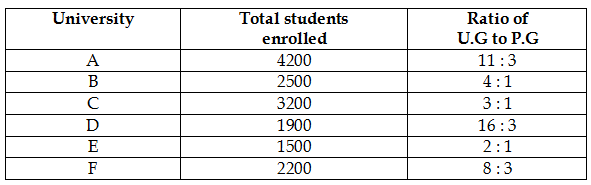## PREPARATORY QUESTIONS

### DATA INTERPRETATION (TABLE)

#### PREPARATORY SET-37 (TABLE)

Direction (Qs.1 to 5): The given table shows the total students enrolled in 6 different universities and ratio of under graduate and post graduate among them. Study the table carefully and answer the following questions.Question No : 1

Find the ratio of students enrolled in PG from University B and D together to students enrolled in U.G from University C & F together.

(1) 3 : 4

(2) 1 : 5

(3) 7 : 11

(4) 13 : 19

(5) 4 : 5

Question No : 2

If 66(2/3)% of P.G. students from University F are female and 12(1/2)% PG students from university C are males. Than females studying in P.G. in University C and F together are what percent of students studying in UG in university C and F together.

(1) 35%

(2) 27.5%

(3) 22%

(4) 13.75%

(5) 25%

Question No : 3

Find the difference in number of students in UG and PG from all the university except university D.

(1) 7000

(2) 6500

(3) 6800

(4) 7200

(5) 6600

Question No : 4

If 30%, 25% and 50% of enrolled student are female in U.G. in University A, B, C respectively and 90%, 60% and 50% PG students are male in universities A, B and C respectively. Then find the total number of females enrolled in university A, B and C.

(1) 3694

(2) 2250

(3) 3380

(4) 2740

(5) 5134

Question No : 5

If 60% of students in U.G. in each university belongs to rural area and 80% of students in P.G. in each university belongs to urban area then find the ratio of total rural students to total urban students in university E & F together.

(1) 89 : 96

(2) 77 : 91

(3) 19 : 31

(4) 13 : 41

(5) 79 : 80